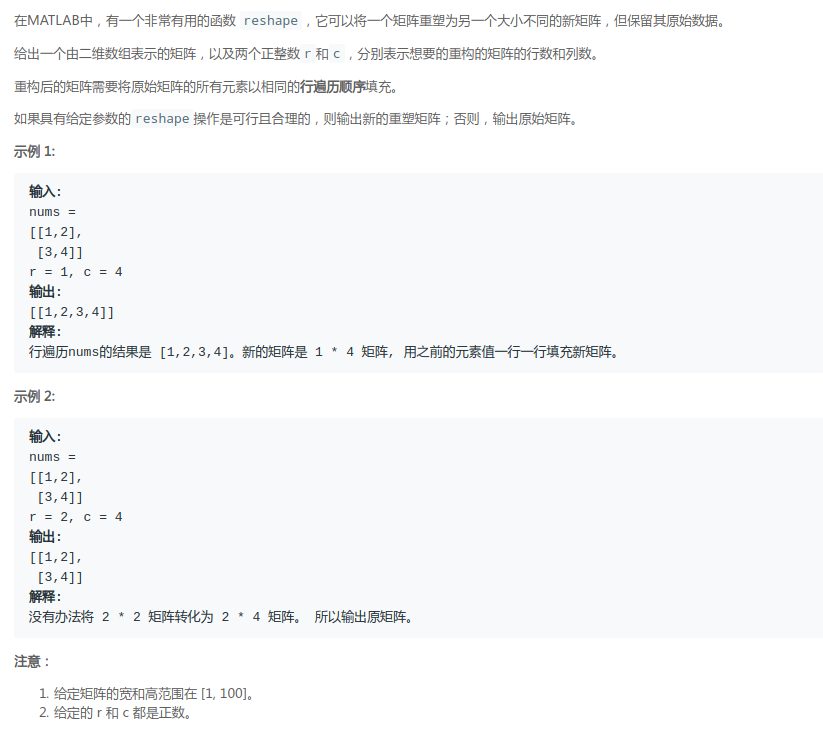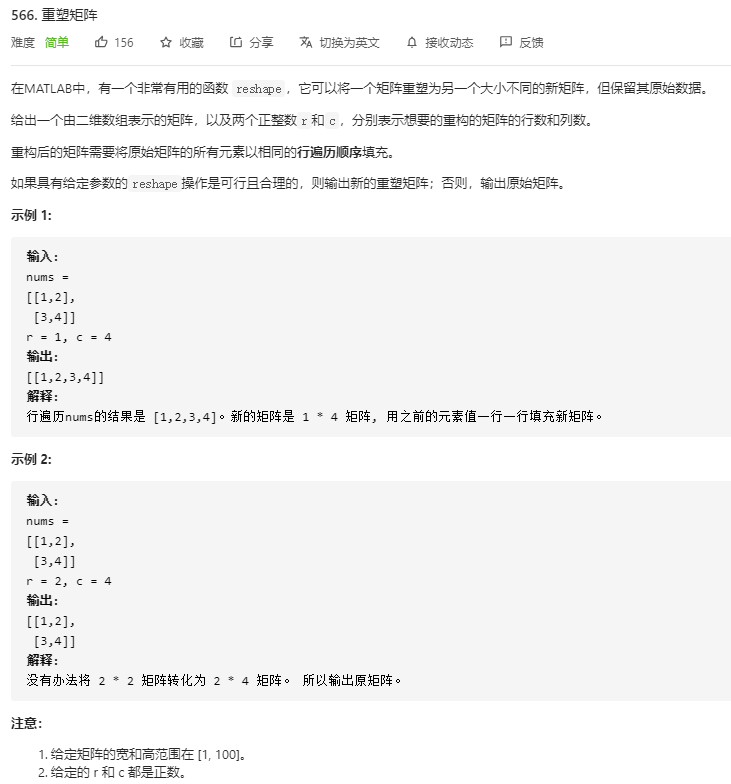• 2021-02-26 18:47:10

# 1 题目描述

在MATLAB中，有一个非常有用的函数 reshape，它可以将一个矩阵重塑为另一个大小不同的新矩阵，但保留其原始数据。
给出一个由二维数组表示的矩阵，以及两个正整数r和c，分别表示想要的重构的矩阵的行数和列数。
重构后的矩阵需要将原始矩阵的所有元素以相同的行遍历顺序填充。
如果具有给定参数的reshape操作是可行且合理的，则输出新的重塑矩阵；否则，输出原始矩阵。

# 2 示例描述

## 2.1 示例 1

输入: nums =
[[1,2],
[3,4]]
r = 1, c = 4
输出: [[1,2,3,4]]
解释:行遍历nums的结果是 [1,2,3,4]。新的矩阵是 1 * 4 矩阵, 用之前的元素值一行一行填充新矩阵。

## 2.2 示例 2

输入: nums =
[[1,2],
[3,4]]
r = 2, c = 4
输出:
[[1,2],
[3,4]]
解释:没有办法将 2 * 2 矩阵转化为 2 * 4 矩阵。 所以输出原矩阵。

# 3 解题提示

给定矩阵的宽和高范围在 [1, 100]。
给定的 r 和 c 都是正数。

暴力求解，干就完了。

# 5 源码详解（C++）

class Solution {
public:
vector<vector<int>> matrixReshape(vector<vector<int>>& nums, int r, int c) {
vector<vector<int>> res( r , vector<int>( c ) ) ;
if ( r * c != ( nums.size() * nums.size() ) )
{
return nums ;
}
for ( int i = 0 ; i < (nums.size() * nums.size()) ; i ++ )
{
res[i / c][i % c] = nums[i / nums.size()][i % nums.size()] ;
}
return res ;
}
};c++ leetcode 数组
更多相关内容
• Reshape the Matrix(重塑矩阵)(简单题) 直接填充 坐标对应 题目链接 题目 解析 直接填充 这个方法很简单，遍历nums中的元素，一个一个填充到新数组即可。 public int[][] matrixReshape(int...

## LeetCode - 566. Reshape the Matrix(重塑矩阵)(简单题)

• 直接填充
• 坐标对应

#### 题目### 解析

#### 直接填充

这个方法很简单，遍历nums中的元素，一个一个填充到新数组即可。

class Solution {

public int[][] matrixReshape(int[][] nums, int r, int c) {
if (nums.length == 0 || nums.length == 0)
return nums;
int n = nums.length;
int m = nums.length;
if (n * m != r * c) return nums;

int[][] res = new int[r][c];
int p = 0, q = 0;
for (int i = 0; i < n; i++) {
for (int j = 0; j < m; j++) {
res[p][q] = nums[i][j];
if (++q == c) {
++p;
q = 0;
}
}
}
return res;
}
}


### 坐标对应

这个是考虑一重循环，找坐标的对应的关系，在矩阵中，一维遍历的nums[i]在二维中横坐标为i/c，纵坐标为i % c，所以可以用一个一重循环遍历。

class Solution {

public int[][] matrixReshape(int[][] nums, int r, int c) {
if (nums.length == 0 || nums.length == 0)
return nums;
int n = nums.length;
int m = nums.length;
if (n * m != r * c)
return nums;
int[][] res = new int[r][c];
for (int i = 0; i < n * m; i++)
res[i / c][i % c] = nums[i / m][i % m];
return res;
}
}


展开全文• 题目 传送门 在MATLAB中，有一个非常有用的函数 ...如果具有给定参数的reshape操作是可行且合理的，则输出新的重塑矩阵；否则，输出原始矩阵。 示例 1: 输入: nums = [[1,2], [3,4]] r = 1, c = 4 输出: [[1,2,3,4]]

## 题目

传送门

在MATLAB中，有一个非常有用的函数 reshape，它可以将一个矩阵重塑为另一个大小不同的新矩阵，但保留其原始数据。
给出一个由二维数组表示的矩阵，以及两个正整数r和c，分别表示想要的重构的矩阵的行数和列数。
重构后的矩阵需要将原始矩阵的所有元素以相同的行遍历顺序填充。
如果具有给定参数的reshape操作是可行且合理的，则输出新的重塑矩阵；否则，输出原始矩阵。

示例 1:

输入:
nums =
[[1,2],
[3,4]]
r = 1, c = 4
输出:
[[1,2,3,4]]
解释:
行遍历nums的结果是 [1,2,3,4]。新的矩阵是 1 * 4 矩阵, 用之前的元素值一行一行填充新矩阵。

示例 2:

输入:
nums =
[[1,2],
[3,4]]
r = 2, c = 4
输出:
[[1,2],
[3,4]]
解释:
没有办法将 2 * 2 矩阵转化为 2 * 4 矩阵。 所以输出原矩阵。

注意：

给定矩阵的宽和高范围在 [1, 100]。
给定的 r 和 c 都是正数。


来源：力扣（LeetCode）

## 解题

### 模板

/**
* Return an array of arrays of size *returnSize.
* The sizes of the arrays are returned as *returnColumnSizes array.
* Note: Both returned array and *columnSizes array must be malloced, assume caller calls free().
* 返回一个大小为*returnSize的数组。
*数组的大小以*returnColumnSizes数组的形式返回。
*注意:返回的数组和*columnSizes数组必须是错置的，假设调用方调用free()
*/
int** matrixReshape(int** nums, int numsSize, int* numsColSize, int r, int c, int* returnSize, int** returnColumnSizes){

}


### 分析题意

我们已知题目的要求就是对于我们输入的原二维数组进行一个变换，判断其是否能够变换成要求的r*c的二维数组，如果可以就输出转换后的二维数组，转换不成功就将原二维数组输出，所以我们需要对于原数组是否能够转换进行判断。

### 分步编码

#### 初始化得到原数组的行数和列数

    int m = numsSize;
int n = numsColSize;


得到后我们需要进行元素个数判断，来决定是否需要进行下一步的转换操作，如果不满足mn=rc，则直接返回原数组即可，同时对于returnSize和returnColumnSizes进行一个简单的和原数组的同步。

    if (m * n != r * c) {
*returnSize = numsSize;
*returnColumnSizes = numsColSize;
return nums;
}


如果满足了元素个数合理就继续转换
首先对于新的r*c数组进行初始化

*returnSize = r;//r行
*returnColumnSizes = malloc(sizeof(int) * r);//初始化分配内存并返回指向可使用的内存地址大小为r个整型数据
int** ans = malloc(sizeof(int*) * r);//同上

for (int i = 0; i < r; i++) {
(*returnColumnSizes)[i] = c;//c列
ans[i] = malloc(sizeof(int) * c);//为每一行的初始指向一可用的内存地址大小为c个整型数据
}


初始化之后就可以对于两个数组之间进行转化；
转化方式很简单我们通过除法和模运算得到两者的对应位置
总元素个数是m*n个，行与列也都是从0开始的，所以x/n就是原二维数组中的第几行，x%n就是原二维数组中的第几列。同理，x/c就是新二维数组中的第几行，x%c就是新二维数组中的第几列

    for (int x = 0; x < m * n; ++x) {
ans[x / c][x % c] = nums[x / n][x % n];
}


最后返回ans即可

### 完整代码

int** matrixReshape(int** nums, int numsSize, int* numsColSize, int r, int c, int* returnSize, int** returnColumnSizes) {
int m = numsSize;
int n = numsColSize;
if (m * n != r * c) {
*returnSize = numsSize;
*returnColumnSizes = numsColSize;
return nums;
}
*returnSize = r;
*returnColumnSizes = malloc(sizeof(int) * r);
int** ans = malloc(sizeof(int*) * r);

for (int i = 0; i < r; i++) {
(*returnColumnSizes)[i] = c;
ans[i] = malloc(sizeof(int) * c);
}
for (int x = 0; x < m * n; ++x) {
ans[x / c][x % c] = nums[x / n][x % n];
}
return ans;
}

展开全文leetcode 算法 数据结构
• 重塑矩阵-C语言 题目描述 解题思路 主要使用的是指针的指针。 代码 int** matrixReshape(int** nums, int numsSize, int* numsColSize, int r, int c, int* returnSize, int** returnColumnSizes){ if (numsSize ...

## LeetCode 566. 重塑矩阵-C语言

题目描述解题思路
主要使用的是指针的指针。

代码

int** matrixReshape(int** nums, int numsSize, int* numsColSize, int r, int c, int* returnSize, int** returnColumnSizes){
if (numsSize * (*numsColSize) != r * c) {
*returnSize = numsSize;
*returnColumnSizes = numsColSize;
return nums;
}
int i, j;
int index = 0;
int colindex = 0;
int **res = (int**)malloc(sizeof(int*) * r);
*returnSize = r;
*returnColumnSizes = (int*)malloc(sizeof(int*) * r);
for (i = 0; i < r; i++) {
res[i] = (int*)malloc(sizeof(int) * c);
(*returnColumnSizes)[i] = c;
}
for (i = 0; i < numsSize; i++) {
for (j = 0; j < *numsColSize; j++) {
if (colindex == c) {
colindex = 0;
index++;
}
res[index][colindex++] = nums[i][j];
}
}
return res;
}展开全文指针 leetcode 数据结构
• 在 MATLAB 中，有一个非常有用的函数 reshape ，它可以将一个m x n 矩阵重塑为另...如果具有给定参数的 reshape 操作是可行且合理的，则输出新的重塑矩阵；否则，输出原始矩阵。 示例 1： 输入：mat = [[1,2],...matlab leetcode
• 在MATLAB中，有一个非常有用的函数 reshape，它可以将一个矩阵重塑为另一个大小不同的新矩阵，...如果具有给定参数的reshape操作是可行且合理的，则输出新的重塑矩阵；否则，输出原始矩阵。示例 1:输入: nums = [...leetcode 数组
• 本题题意很简单，直接遍历矩阵即可 代码如下： #include #include #include #include #include #include #include #include #include #include #include #include #...
• 题目描述：在MATLAB中，有一个非常有用的函数 reshape，它可以将一个矩阵重塑为另一个大小不同的新...如果具有给定参数的reshape操作是可行且合理的，则输出新的重塑矩阵；否则，输出原始矩阵。示例 1:输入: nums...
• 否则，对于 x∈[0,m * n)，第 x个元素在 nums 中对应的下标为 (x /n, x% n)，而在新的重塑矩阵中对应的下标为(x / c,x % c)。我们直接进行赋值即可。 代码 class Solution { public int[][] matrixReshape(int[][] ...leetcode 算法
• 在MATLAB中，有一个非常有用的函数 reshape，它可以将一个矩阵重塑为另一个大小不同的新矩阵，但保留...如果具有给定参数的reshape操作是可行且合理的，则输出新的重塑矩阵；否则，输出原始矩阵。 示例 1: 输入:...
• 在MATLAB中，有一个非常有用的函数 reshape，它可以将一个矩阵重塑为另一个大小不同的新矩阵，但保留其原始...如果具有给定参数的reshape操作是可行且合理的，则输出新的重塑矩阵；否则，输出原始矩阵。 示例 1: ...
• 《坐标转换》简单01 —— LeetCode 566. 重塑矩阵c++ 算法 数据结构 取模
• 我们需要使用C语言来创建一个动态的二维数组，使它每行的列数等于它的行数。 首先通过malloc()函数来开辟整个二维数组的空间： malloc()函数： malloc函数会返回开辟空间的首地址，加(int *)的目的是让计算机知道，...leetcode
• 566. 重塑矩阵 /** * Return an array of arrays of size *returnSize. * The sizes of the arrays are returned as *returnColumnSizes array. * Note: Both returned array and *columnSizes array must be ...算法
• 【leetcode】#566 Reshape the Matrix 重塑矩阵 改变矩阵维度 C语言中 matColSize 的含义 在MATLAB中，有一个非常有用的函数 reshape，它可以将一个矩阵重塑为另一个大小不同的新矩阵，但保留其原始数据。 给出一个...leetcode
• 题目： 在MATLAB中，有一个非常有用的函数 ...如果具有给定参数的reshape操作是可行且合理的，则输出新的重塑矩阵；否则，输出原始矩阵。 示例 1: 输入: nums = [[1,2], [3,4]] r = 1, c = 4 输出: [[1,2,3,...leetcode arraylist
• 【LeetCode】第566题——重塑矩阵（难度：简单）题目描述解题思路代码详解思路一：双循环，下标+1检索思路二：双循环，下标取整取余检索注意点 题目描述 在MATLAB中，有一个非常有用的函数 reshape，它可以将一个...leetcode java
• 题目描述 在MATLAB中，有一个非常有用的函数 reshape，它可以将一个矩阵重塑为另一个大小不同的新矩阵，...如果具有给定参数的reshape操作是可行且合理的，则输出新的重塑矩阵；否则，输出原始矩阵。 示例 1: 输入:...python leetcode
• 重塑矩阵 /** * Return an array of arrays of size *returnSize. * The sizes of the arrays are returned as *returnColumnSizes array. * Note: Both returned array and *columnSizes array must be malloced, ...
• 反思 想到的方法和官方答案一样，即矩阵重塑时，使用的双下标与元素数i、列数c有关： 第i个元素的行数是(i - 1)/c,当元素从k=0开始遍历时为k/c 第i个元素的列数是（i%c）- 1,当元素从k=0开始遍历时为k%c 还有一个新...c++
• 颜色分类-C语言实现-中等难度题题目传送门给定一个包含红色、白色和蓝色，一共 n 个元素的数组，原地对它们进行排序，使得相同颜色的元素相邻，并按照红色、白色、蓝色顺序排列。此题中，我们使用整数 0、 1 和 2 ...
• 本系列文章为浙江大学翁恺C语言程序设计学习笔记，前面的系列文章链接如下： C语言程序设计学习笔记：P1-程序设计与C语言 C语言程序设计学习笔记：P2-计算 C语言程序设计学习笔记：P3-判断 C语言程序设计学习笔记：...代码规范 编程语言 算法
• [转]Python中的矩阵转置 Python中的矩阵转置 via 需求: 你需要转置一个二维数组,将行列互换. 讨论: 你需要确保该数组的行列数都是相同的.比如: arr = [[1, 2, 3], [4, 5, 6], [7, 8 ... 【python】bytearray和......